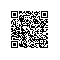# java泛型 通配符详解及实践

java泛型,你想知道的一切

## 一个问题代码

    public static void main(String[] args) {
// 编译报错
// required ArrayList<Integer>, found ArrayList<Number>
ArrayList<Integer> list1 = new ArrayList<>();
ArrayList<Number> list2 = list1;

// 可以正常通过编译,正常使用
Integer[] arr1 = new Integer[]{1, 2};
Number[] arr2 = arr1;
}


## 不变,协变,逆变的定义

• f(⋅)是逆变（contravariant）的，当A≤B时有f(B)≤f(A)成立；**
• f(⋅)是协变（covariant）的，当A≤B时有f(A)≤f(B)成立；**
• f(⋅)是不变（invariant）的，当A≤B时上述两个式子均不成立，即f(A)与f(B)相互之间没有继承关系**

## 通配符

? extends T(上边界通配符upper bounded wildcard) 对应协变关系,表示 ? 是继承自 T的任意子类型.也表示一种约束关系,只能提供数据,不能接收数据.

? 的默认实现是 ? extends Object, 表示 ? 是继承自Object的任意类型.

? super T(下边界通配符lower bounded wildcard) 对应逆变关系,表示 ?T的任意父类型.也表示一种约束关系,只能接收数据,不能提供你数据.

    public static void main(String[] args) {
ArrayList<Integer> list1 = new ArrayList<>();
// 协变, 可以正常转化, 表示list2是继承 Number的类型
ArrayList<? extends Number> list2 = list1;

// 无法正常添加
// ? extends Number 被限制为 是继承 Number的任意类型,
// 可能是 Integer,也可能是Float,也可能是其他继承自Number的类,
// 所以无法将一个确定的类型添加进这个列表,除了 null之外
list2.add(new Integer(1));
// 可以添加
list2.add(null);

// 逆变
ArrayList<Number> list3 = new ArrayList<>();
ArrayList<? super Number> list4 = list3;
list4.add(new Integer(1));
}


### ? 与 T 的差别

1. ? 表示一个未知类型, T 是表示一个确定的类型. 因此,无法使用 ?T 声明变量和使用变量.如
    // OK
static <T> void test1(List<T> list) {
T t = list.get(0);
t.toString();
}
// Error
static void test2(List<?> list){
? t = list.get(0);
t.toString();
}java

1. ? 主要针对 泛型类的限制, 无法像 T类型参数一样单独存在.如
    // OK
static <T> void test1(T t) {
}
// Error
static void test2(? t){
}

1. ? 表示 ? extends Object, 因此它是属于 in类型(下面会说明),无法接收数据, 而T可以.
    // OK
static <T> void test1(List<T> list, T t) {
list.add(t);
}
// Error
static void test2(List<?> list, Object t) {
list.add(t);
}

1. ? 主要表示使用泛型,T表示声明泛型

// Error
public class Holder<?> {
...
// OK
public class Holder<T> {
...
public static void main(String[] args) {
// OK
Holder<?> holder;
// Error
Holder<T> holder;
}

1. 永远不要在方法返回中使用?,在方法中不会报错,但是方法的接收者将无法正常使用返回值.因为它返回了一个不确定的类型.

## 通配符的使用准则

"in"类型:
“in”类型变量向代码提供数据。 如copy（src，dest） src参数提供要复制的数据，因此它是“in”类型变量的参数。

"out"类型:
“out”类型变量保存接收数据以供其他地方使用.如复制示例中，copy（src，dest），dest参数接收数据，因此它是“out”参数。

### "in","out" 准则

• "in" 类型使用 上边界通配符? extends.
• "out" 类型使用 下边界通配符? super.
• 如果即需要 提供数据(in), 又需要接收数据(out), 就不要使用通配符.

    public static <T> void copy(List<? super T> dest, List<? extends T> src) {
...
if (srcSize < COPY_THRESHOLD ||
(src instanceof RandomAccess && dest instanceof RandomAccess)) {
for (int i=0; i<srcSize; i++)
// dest 接收数据, src 提供数据
dest.set(i, src.get(i));
}
...
}


### PECS(producer-extends,consumer-super)

get属于生产者,put属于消费者. 这样的概念比较难懂.

// dest 消费了数据(set),则使用 super
// src 生产了数据(get), 则使用 extends
dest.set(i, src.get(i));


## 动手编写通配符函数

1. map() 函数

strArr = ["1", "2"]
intArr = map(lambda x: int(x) * 10, strArr)
print(strArr,list(intArr))
# ['1', '2'] [10, 20]


public class Main {
public static void main(String[] args) {
List<String> strList = new ArrayList<>();
strList.add("1");
strList.add("2");
// jdk8 使用lambda表达式
List<Integer> intList = map(strList, s -> Integer.parseInt(s) * 10);
// strList["1","2"]
// intList[10,20]
}

/**
* 定义一个接口,它接收一个类型,返回另一个类型.
*
* @param <T> 一个类型的方法参数
* @param <R> 一个类型的返回
*/
interface Func_TR<T, R> {
// 接收一个类型,返回另一个类型.
R apply(T t);
}

/**
* 定义mapping函数
*
* @param src    提供数据,因此这里使用(get) 上边界通配符
* @param mapper mapping 函数的具体实现
* @param <?     extends R> 提供数据,这里是作为apply的返回值, 因此使用 上边界通配符
* @param <?     super T>接收数据,这里作为 apply的传入参数
* @return 返回值不要使用 通配符来定义
*/
public static <R, T> List<R> map(List<? extends T> src, Func_TR<? super T, ? extends R> mapper) {
if (src == null)
throw new IllegalArgumentException("List must not be not null");
if (mapper == null)
throw new IllegalArgumentException("map func must be not null");
// coll 既需要接收数据(add),又需要提供数据(return),所以不使用通配符
List<R> coll = new ArrayList<>();
for (T t : src) {
coll.add(mapper.apply(t));
}
return coll;
}

1. filter() 函数

Python中,filter() 函数用于过滤序列，过滤掉不符合条件的元素，返回由符合条件元素组成的新列表。

intArr = [1, 2, 3, 4, 5]
newArr = filter(lambda x: x >= 3, intArr)
print(list(newArr))
# [1, 2, 3, 4, 5] [3, 4, 5]


public class Main {
public static void main(String[] args) {
List<Integer> intList = new ArrayList<>();
intList.add(1);
intList.add(2);
intList.add(3);
intList.add(4);
intList.add(5);

List<Integer> filterList = filter(intList, i -> i >= 3);
// filterList[3,4,5]
}
/**
* 定义一个接口,它接收一个类型,返回布尔值
*
* @param <T> 一个类型的方法参数
*/
interface Func_Tb<T> {
boolean apply(T t);
}

/**
* filter 函数的实现
*
* @param src  传入的列表只提供数据,这里只调用了迭代操作, 因此使用 上边界通配符
* @param func func需要接收一个数据,  因此使用 下边界通配符
* @return 返回值不要使用 通配符来定义,返回过滤后的列表
*/
public static <T> List<T> filter(List<? extends T> src, Func_Tb<? super T> func) {
if (src == null)
throw new IllegalArgumentException("List must not be not null");
if (func == null)
throw new IllegalArgumentException("filter func must be not null");

// coll 既需要接收数据(add),又需要提供数据(return),所以不使用通配符
List<T> coll = new ArrayList<>();
for (T t : src) {
if (func.apply(t))
coll.add(t);
}
return coll;
}
}

1. reduce()函数

Python中,reduce() 函数会对参数序列中元素进行累积。

from functools import reduce
result = reduce(lambda x, y: x + y, [1, 2, 3, 4, 5])
print(result)
# 15


public class Main {
public static void main(String[] args) {
List<Integer> intList = new ArrayList<>();
intList.add(1);
intList.add(2);
intList.add(3);
intList.add(4);
intList.add(5);

int result = reduce(intList, (t1, t2) -> t1 + t2);
// result = 15
}
/**
* 定义一个接口,接收两个同一个类型的参数,返回值也属于同一类型
*
* @param <T> 作为方法参数,和返回值
*/
interface Func_TTT<T> {
T apply(T t1, T t2);
}

/**
* reduce函数的实现
*
* @param src  传入的列表只提供数据,这里只调用了迭代操作, 因此使用 上边界通配符
* @param func T 作为 apply()函数的参数和返回值,即接收也提供数据, 因此不能使用通配符
* @return 返回值不要使用 通配符来定义, 返回参数相互迭代的值
*/
public static <T> T reduce(List<? extends T> src, Func_TTT<T> func) {
if (src == null || src.size() == 0)
throw new IllegalArgumentException("List must not be not null or empty");
if (func == null)
throw new IllegalArgumentException("reduce func must be not null");

int size   = src.size();
T   result = src.get(0);
if (size == 1) return result;
// 将前两项的值做apply操作后的返回值,再与下一个元素进行操作
for (int i = 1; i < size; i++) {
T ele = src.get(i);
result = func.apply(result, ele);
}
return result;
}
}
`

## 参考使用钉钉扫一扫加入圈子
+ 订阅